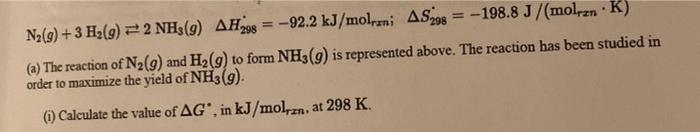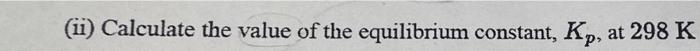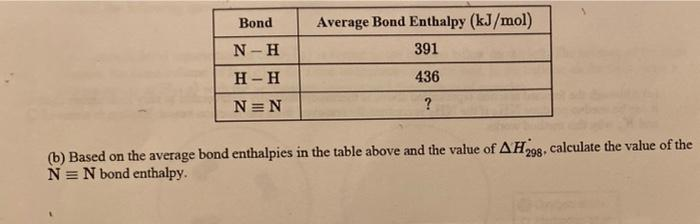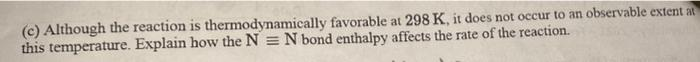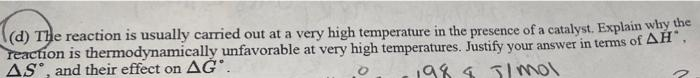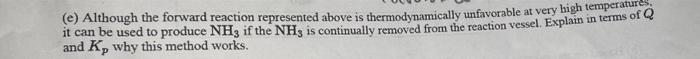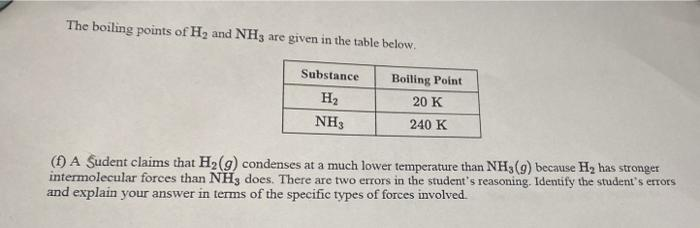# Question N2(g) + 3 Hz(9) = 2 NH3(9) AH = -92.2 kJ/molzni AS208 -198.8 J/(molranK) (a) The reaction of N2(9) and H2(g) to form NH3(9) is represented above. The reaction has been studied in order to maximize the yield of NH3(g). () Calculate the value of AG, in kJ/molzn, at 298 K. (ü) Calculate the value of the equilibrium constant, Kp, at 298 K Bond Average Bond Enthalpy (kJ/mol) 391 N-H 436 H-H NEN ? (b) Based on the average bond enthalpies in the table above and the value of AH298. calculate the value of the N= N bond enthalpy. (c) Although the reaction is thermodynamically favorable at 298 K, it does not occur to an observable extent a this temperature. Explain how the N = N bond enthalpy affects the rate of the reaction. (d) The reaction is usually carried out at a very high temperature in the presence of a catalyst. Explain why the Teaction is thermodynamically unfavorable at very high temperatures. Justify your answer in terms of AH", AS, and their effect on AĞ'. 198 a Imol () Although the forward reaction represented above is thermodynamically unfavorable at very high temperas of Q it can be used to produce NH3 if the NH, is continually removed from the reaction vessel. Explain in terms of Q and Ky why this method works. The boiling points of H2 and NH3 are given in the table below. Substance H2 NH Boiling Point 20 K 240 K (1) A Sudent claims that H2(g) condenses at a much lower temperature than NH (9) because H, has stronger intermolecular forces than NH3 does. There are two errors in the student's reasoning. Identify the student's errors and explain your answer in terms of the specific types of forces involved